Practice Test: Civil Engineering (CE)- 5

# Practice Test: Civil Engineering (CE)- 5

Test Description

## 65 Questions MCQ Test GATE Civil Engineering (CE) 2023 Mock Test Series | Practice Test: Civil Engineering (CE)- 5

Practice Test: Civil Engineering (CE)- 5 for Civil Engineering (CE) 2023 is part of GATE Civil Engineering (CE) 2023 Mock Test Series preparation. The Practice Test: Civil Engineering (CE)- 5 questions and answers have been prepared according to the Civil Engineering (CE) exam syllabus.The Practice Test: Civil Engineering (CE)- 5 MCQs are made for Civil Engineering (CE) 2023 Exam. Find important definitions, questions, notes, meanings, examples, exercises, MCQs and online tests for Practice Test: Civil Engineering (CE)- 5 below.
Solutions of Practice Test: Civil Engineering (CE)- 5 questions in English are available as part of our GATE Civil Engineering (CE) 2023 Mock Test Series for Civil Engineering (CE) & Practice Test: Civil Engineering (CE)- 5 solutions in Hindi for GATE Civil Engineering (CE) 2023 Mock Test Series course. Download more important topics, notes, lectures and mock test series for Civil Engineering (CE) Exam by signing up for free. Attempt Practice Test: Civil Engineering (CE)- 5 | 65 questions in 180 minutes | Mock test for Civil Engineering (CE) preparation | Free important questions MCQ to study GATE Civil Engineering (CE) 2023 Mock Test Series for Civil Engineering (CE) Exam | Download free PDF with solutions
 1 Crore+ students have signed up on EduRev. Have you?
Practice Test: Civil Engineering (CE)- 5 - Question 1

### Question 1 to 5 carry One Mark each Q. Even as the ________else where in the world are struggling, to come out of recession, Indian consumers are splurging on consumer goods and to ____________this growth, companies are investing heavily in various sectors.

Practice Test: Civil Engineering (CE)- 5 - Question 2

### In the olympic games, the flags of six nations were flown on the masts in the following way. T he flag of America was to the left of Indian tricolor and to the right of the flag of france. T he flag of Australia was on the right of the Indian flag but was to the left of the flag of Japan, which was to the left of the flag of China. T he two flags which are in the centre is

Practice Test: Civil Engineering (CE)- 5 - Question 3

### From the given equilateral triangle below A, B and C run along the sides in clockwise direction and stop after coming (1{1over 2}) sides. Which of the following statements is true?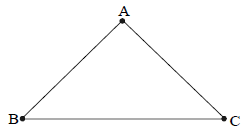Detailed Solution for Practice Test: Civil Engineering (CE)- 5 - Question 3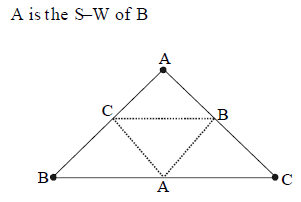Practice Test: Civil Engineering (CE)- 5 - Question 4

In the following questions, a sent ence has been given in direct/indirect speech.
Nitin said to me, “why don’t you come with us? why do you want to be so unsocial.

Practice Test: Civil Engineering (CE)- 5 - Question 5

Select the grammatical error part.

In order to transfer (a)/ branch licenses for one (b)/ bank to anot her you require special approval from RBI
(c)/ No error (d).

Practice Test: Civil Engineering (CE)- 5 - Question 6

Question 6 to 10 carry Two Marks each

Q.

In an NGO, t he daily average wages of 20 illiterate employee is decreased from Rs. 25 to Rs. 10. T hus the
average salary of all the literate (educated) and illiterate employees is decreased by Rs. 10 perday. T he
number of educated employees working in the NGO is

Practice Test: Civil Engineering (CE)- 5 - Question 7

Directi on for questi ons (7-8).
Answer the question with the help of the following table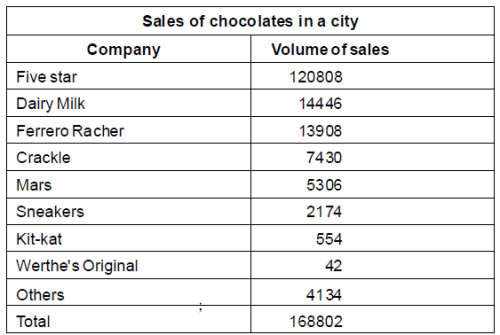Q.

The ratio of the percentage share of Dairy Milk to that of other is

Detailed Solution for Practice Test: Civil Engineering (CE)- 5 - Question 7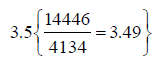Practice Test: Civil Engineering (CE)- 5 - Question 8

Answer the question with the help of the following tableQ.

If in the next year, Crackle and Dairy Mill decided to join hands and increase their market share from their
combined existing share by 20%. What will be their combined production if the mark size remains the same?

Detailed Solution for Practice Test: Civil Engineering (CE)- 5 - Question 8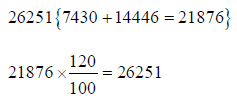Practice Test: Civil Engineering (CE)- 5 - Question 9

Simple interest on a certain amount is 9/16  of the principle. If the number represent ing the rate of intersest in percent and time in years be equal. Then time, for when the principal is lent out is,

Detailed Solution for Practice Test: Civil Engineering (CE)- 5 - Question 9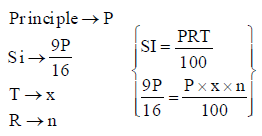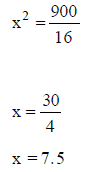Practice Test: Civil Engineering (CE)- 5 - Question 10

If A is a square matrix of order 3 and |A| =1/2. The A (a dj(A)) is

Detailed Solution for Practice Test: Civil Engineering (CE)- 5 - Question 10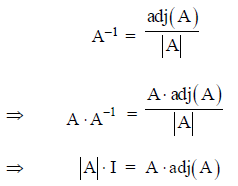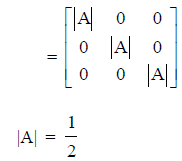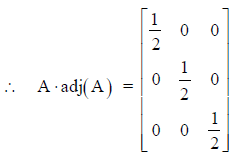Practice Test: Civil Engineering (CE)- 5 - Question 11

Question 11 to 35 carry One Mark each

Q.

If the probability for A to fail in an examination is 0.2 and that for B is 0.3, then the probability that either A or B fails is

Detailed Solution for Practice Test: Civil Engineering (CE)- 5 - Question 11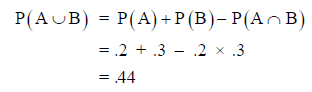Practice Test: Civil Engineering (CE)- 5 - Question 12

In a plastic hinge, the actual distribution of strain across the sector will be as in

Detailed Solution for Practice Test: Civil Engineering (CE)- 5 - Question 12

The eigen value of unitary matrix are always of unit modulus

Practice Test: Civil Engineering (CE)- 5 - Question 13

According to coulamb’s wedge theory the active earth pressure whcih slides the wedge acts

Detailed Solution for Practice Test: Civil Engineering (CE)- 5 - Question 13

Coulomb’s theory of earth pressure involves the consideration of sliding wedge which tends to brreak away from the rest of the backfill upon wall movement, in active earth pressure wall moves outward, the sliding wedge moves downwards and outward.

Practice Test: Civil Engineering (CE)- 5 - Question 14

If 'u' and 'v' the component of velocity in x-direction and y-direction respectively are given u = ax+by and
v = cx + dy then the condition to be satisfy is

Detailed Solution for Practice Test: Civil Engineering (CE)- 5 - Question 14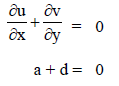Practice Test: Civil Engineering (CE)- 5 - Question 15

Eigen value of unitary matrix are always

Detailed Solution for Practice Test: Civil Engineering (CE)- 5 - Question 15

The eigen value of unitary matrix are always of unit modulus

Practice Test: Civil Engineering (CE)- 5 - Question 16

Method consistent deformation is based on

*Answer can only contain numeric values
Practice Test: Civil Engineering (CE)- 5 - Question 17

Presence of ifluoride in water greater than permissible level of 1.5 mg/l causes

Detailed Solution for Practice Test: Civil Engineering (CE)- 5 - Question 17

Nearly 22 % of groundwater has more than the permissible limit of fluoride (1.5 mg L−1), which is responsible for the endemic dental fluorosis in the area concerned.

*Answer can only contain numeric values
Practice Test: Civil Engineering (CE)- 5 - Question 18

If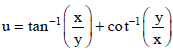, then the value of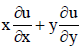is

Detailed Solution for Practice Test: Civil Engineering (CE)- 5 - Question 18

If, then the value ofis

Practice Test: Civil Engineering (CE)- 5 - Question 19

The slope area method is extensively used in

Practice Test: Civil Engineering (CE)- 5 - Question 20

A flexible material for D.P.C is

*Answer can only contain numeric values
Practice Test: Civil Engineering (CE)- 5 - Question 21

Jackson turbidity meter is used for water samples having turbidity not less than

_____________________ unit

*Answer can only contain numeric values
Practice Test: Civil Engineering (CE)- 5 - Question 22

The modulus part of (1 + i)8 is

Detailed Solution for Practice Test: Civil Engineering (CE)- 5 - Question 22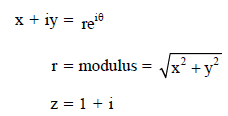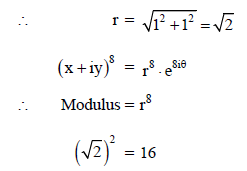Practice Test: Civil Engineering (CE)- 5 - Question 23

The minimum grade of reinforced concrete required for extreme exposure condition is

Practice Test: Civil Engineering (CE)- 5 - Question 24

Geostationary satellites are

Practice Test: Civil Engineering (CE)- 5 - Question 25

If a vector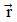is defined as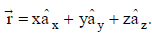The divergenc e of vector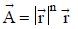is

Detailed Solution for Practice Test: Civil Engineering (CE)- 5 - Question 25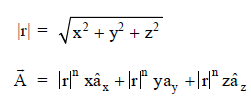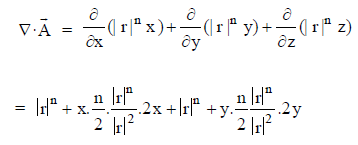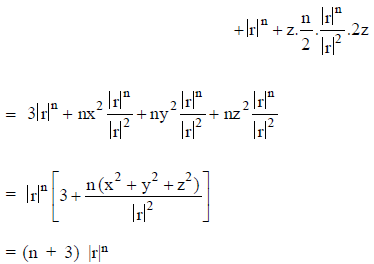*Answer can only contain numeric values
Practice Test: Civil Engineering (CE)- 5 - Question 26

For a road with a camber 6.5 % and design speed of 72 kmph, the minimum radius of the curve beyond
which no super elevation is needed _____________ m.

Detailed Solution for Practice Test: Civil Engineering (CE)- 5 - Question 26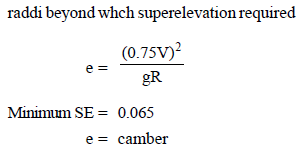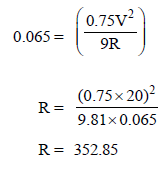*Answer can only contain numeric values
Practice Test: Civil Engineering (CE)- 5 - Question 27

The analysis of maximum daily rainfall in a city indicated that a depth of 280 mm has a return period of
50 year. the probability of one day rainfall depth equal or greater than 280 mm in the city occuring two
times in 15 successive years is ______________

*Answer can only contain numeric values
Practice Test: Civil Engineering (CE)- 5 - Question 28

A beam AB is fixed at A and simply supported at B. A internal hinge is present at C. Deflection at the internal hinge when a anti clockwise moment 30 kNm is applied at B is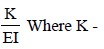(upto two digits after decimal )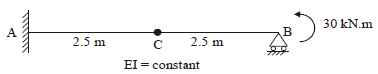____________________

Practice Test: Civil Engineering (CE)- 5 - Question 29

Standard reagent used for testing the turbidity by Nephlometer is

*Answer can only contain numeric values
Practice Test: Civil Engineering (CE)- 5 - Question 30

The maximum height of a low gravity dam of elementary profile made of concrete of relative density 2.45
and safe allowable stress of foundation material 4.16 MPa without considering uplift force is about

Practice Test: Civil Engineering (CE)- 5 - Question 31

A rigid wheel 1.25 diameter is to be provide with a thin steel tyre. If the stress in the steel tyre is not to exceed 140 N/mm2, what is the internal diameter of the tyre? Take E = 2 × 105 N/mm2 and α = 1.2 × 10-5 per oC

Detailed Solution for Practice Test: Civil Engineering (CE)- 5 - Question 31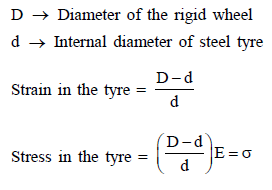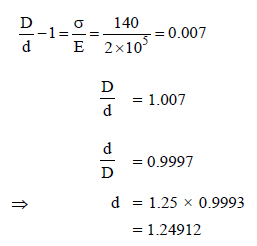*Answer can only contain numeric values
Practice Test: Civil Engineering (CE)- 5 - Question 32

The differemce b/w maximum and minimum void ratio of a sand sample is 0.30. if the relative density of this
sample is 66.6% at a void ratio of 0.40, then the void ratio of this sample at its lossest state will be

Detailed Solution for Practice Test: Civil Engineering (CE)- 5 - Question 32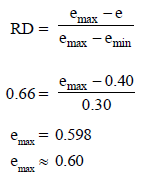Practice Test: Civil Engineering (CE)- 5 - Question 33

Match List-I and List-II and select the correct answer (moment of inertia of solid circular disc) using the
code given below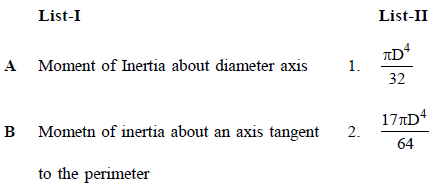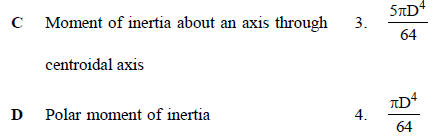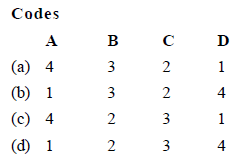Practice Test: Civil Engineering (CE)- 5 - Question 34

After performing the test by di-ionic tester it is found that specific conductance of water sample at 25°C
is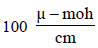. The total dissolved solids in the sample present is

*Answer can only contain numeric values
Practice Test: Civil Engineering (CE)- 5 - Question 35

An overhang beam ABCD is supported at B & C where AB and CD are overhangs. Beam is loaded as
shown below. The load P for which there is no rotation at supports will be kN (upto two digits after decimal)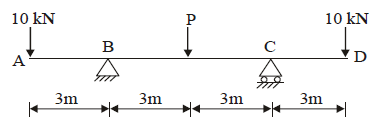*Answer can only contain numeric values
Practice Test: Civil Engineering (CE)- 5 - Question 36

Question 36 to 65 carry Two Marks each

Q.

A single-riveted double cover butt joint is used to connect two plates 16 mm thick with chain riveting. The
rivets used are power driven 20 mm is diameter at a pitch of 60 mm. The efficiency of the joint is

_________________ %.

Detailed Solution for Practice Test: Civil Engineering (CE)- 5 - Question 36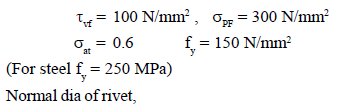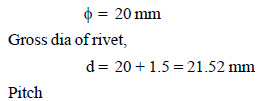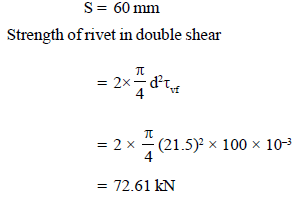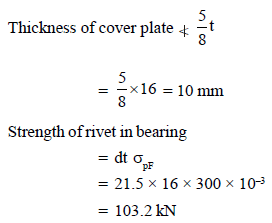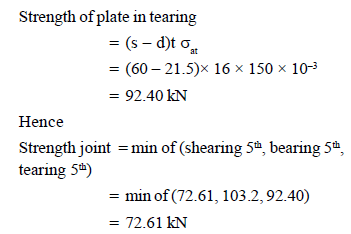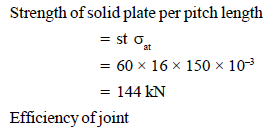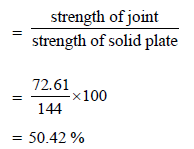Practice Test: Civil Engineering (CE)- 5 - Question 37

If a permeability of soil changes at a certain interface, from k1 to k2 on other interface. the flow line makes
angle of θ1 adn θ2 with normal to the interface the ratio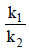is directly proportional to

*Answer can only contain numeric values
Practice Test: Civil Engineering (CE)- 5 - Question 38

Coefficient of static friction between A and the horizontal surface is 0.20. The minimum mass of C to
prevent horizontal sliding is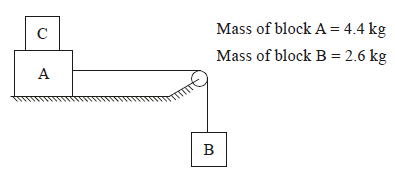(upto one significant digit after decimal)

______________________

*Answer can only contain numeric values
Practice Test: Civil Engineering (CE)- 5 - Question 39

A weir accross an alluvial river has a horizontal floor length 60m and retain 6.5 m of water under full
reserviour condition. If the downstream sheet pile is driven to a depth of 6.5 m below an average bed
level, the exit gradient for this case.

__________________

*Answer can only contain numeric values
Practice Test: Civil Engineering (CE)- 5 - Question 40

What should be the minimum length two a high strength steel rod of 12.5 mm diameter so that one end can be twisted by 30o with respect of the other end, without exceeding a shear stress of 270 N/mm2 given
modulus of rigidity G = 8 × 104 N/mm2

_________________________ m

Detailed Solution for Practice Test: Civil Engineering (CE)- 5 - Question 40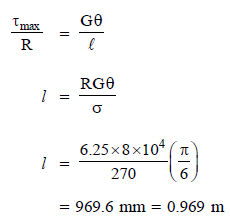*Answer can only contain numeric values
Practice Test: Civil Engineering (CE)- 5 - Question 41

For a site consisting of 1m sand layer underlain by 6m clay resting on rock. fill is constructed using this soil is the 50% consolidation of soil takes place in 6.84 years. Then the time required for 90% consolidation of soil is years _______________

Detailed Solution for Practice Test: Civil Engineering (CE)- 5 - Question 41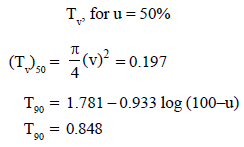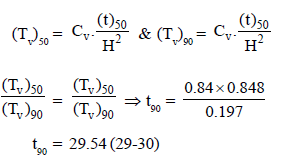Practice Test: Civil Engineering (CE)- 5 - Question 42

Consider the statements

(i) CO2 in water may increase acidity
(ii) CO2 in water may increase alkalinity in the presence of algee in water
Which of the following is correct

*Answer can only contain numeric values
Practice Test: Civil Engineering (CE)- 5 - Question 43

Degree of static interminacy of following structure is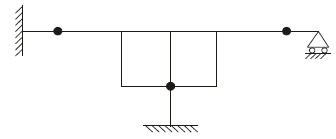___________________

*Answer can only contain numeric values
Practice Test: Civil Engineering (CE)- 5 - Question 44

A clay layer 5m thick, is covered by a 10mn sandy gravel stratum with porority of 30% and with a unit weight
19 kN/m3. Test on undrained sample of clay gave results as follows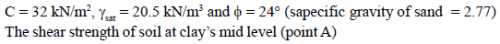____________________ kN/m2

Detailed Solution for Practice Test: Civil Engineering (CE)- 5 - Question 44

Given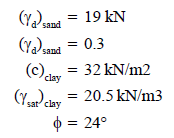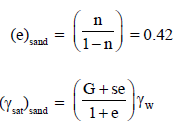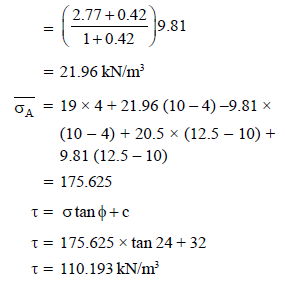*Answer can only contain numeric values
Practice Test: Civil Engineering (CE)- 5 - Question 45

For a highway in plane terrain, if ruling gradient is 1 in 20. the compensated gradient for a curve of radius
120 m is _______________ %

Detailed Solution for Practice Test: Civil Engineering (CE)- 5 - Question 45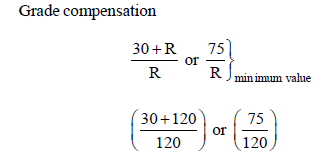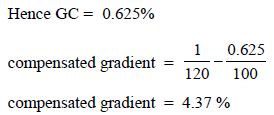*Answer can only contain numeric values
Practice Test: Civil Engineering (CE)- 5 - Question 46

Using Sim pson’s 1/3rd rule with step size of 0.1, the value of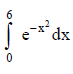is___________

Detailed Solution for Practice Test: Civil Engineering (CE)- 5 - Question 46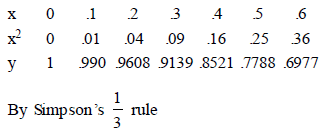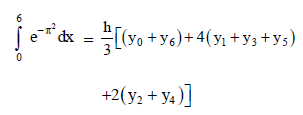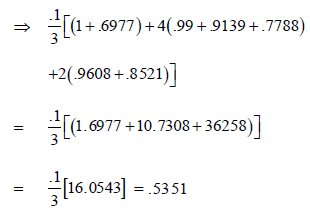Practice Test: Civil Engineering (CE)- 5 - Question 47

For the system Shown as following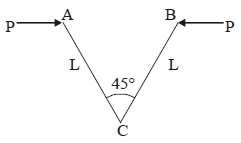Strain energy of the system is

Practice Test: Civil Engineering (CE)- 5 - Question 48

An undrained triaxial test was carried on an undistribed sample of sand with cell pressure of 200 kN/m2. The additional axial load of failure is 342kN. The sample is originally 76mm long and 33mm in diameter is normal stress (σn) at 6m depth is 100 kN/m2 and vertical deformation is 5.1mm then the shear strength in (kN/m2) of sample is

Practice Test: Civil Engineering (CE)- 5 - Question 49

A cantilever beam of span 6m carries a point load of 100 kN at its free end and another point load 'W' at the
mid of its length. If the maximum bending moment is stated to be 900 kNm, the load 'W' will have the value

Detailed Solution for Practice Test: Civil Engineering (CE)- 5 - Question 49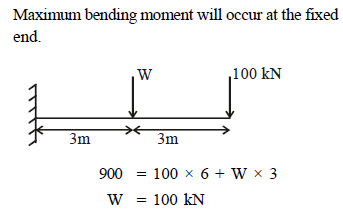Practice Test: Civil Engineering (CE)- 5 - Question 50

A bubble tube has a sensitiveness of 23 seconds for 2 mm division, a staff held 100 m away the error

Detailed Solution for Practice Test: Civil Engineering (CE)- 5 - Question 50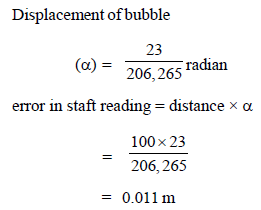Practice Test: Civil Engineering (CE)- 5 - Question 51

The solution of the differential equation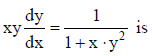Detailed Solution for Practice Test: Civil Engineering (CE)- 5 - Question 51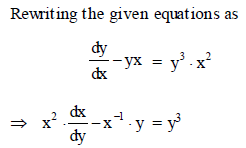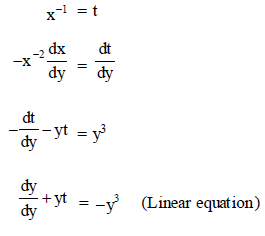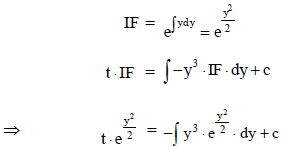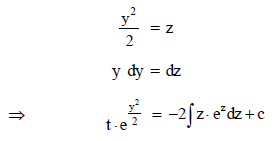*Answer can only contain numeric values
Practice Test: Civil Engineering (CE)- 5 - Question 52

Hourly rainfall of 40, 60 and 80mm occur over a 25 ha area with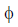= 50 mm/h for 4 ha,= 30 mm/h
for 10 ha and= 10 mm/h for 11 ha. The total rainfall excess for 3h storm will be

________________

Detailed Solution for Practice Test: Civil Engineering (CE)- 5 - Question 52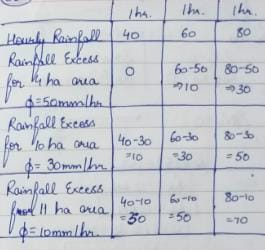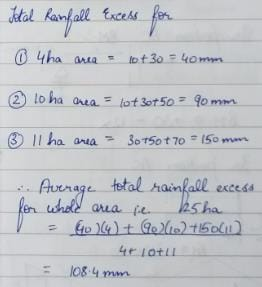Practice Test: Civil Engineering (CE)- 5 - Question 53

Which of the following statement is/are correct
1. Mastic asphalt has self heating property
2. Mastic asphlt contains more percentage of bitumen
3. Synthetic geotextiles are used to make mastic asphalt
4. mastic asphalt is used in heavy duty area

Practice Test: Civil Engineering (CE)- 5 - Question 54

A 60 mm diameter shaft transmitting a torque of 4 kNm is also subjected to an axial tension of 180 kN. The
maximum tensile stress is

Detailed Solution for Practice Test: Civil Engineering (CE)- 5 - Question 54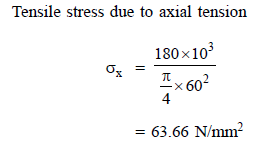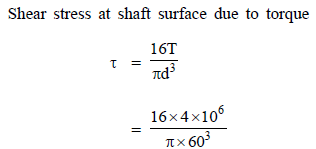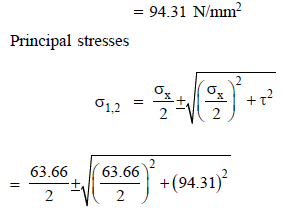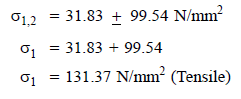Practice Test: Civil Engineering (CE)- 5 - Question 55

What is the tensile strength of a roof tress diagonal 100 × 75 × 10 mm (fy = 260 N/mm2) connected to
a gusset plate if 20 mm diameter popower driven rivet in one row along the length of member. The short
leg of the angle is kep outstanding.

Detailed Solution for Practice Test: Civil Engineering (CE)- 5 - Question 55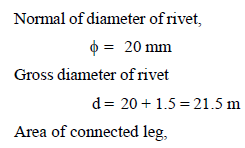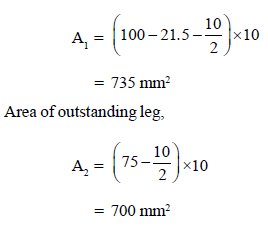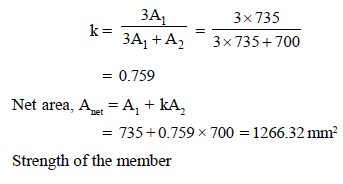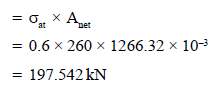*Answer can only contain numeric values
Practice Test: Civil Engineering (CE)- 5 - Question 56

In the measurement of alkalinity if the volume of 0.02 N H2SO4 used is 25 ml then alkalinity of the sample
represented as CaCO3 is (volume of sample is 5l)

______________mg/L

Practice Test: Civil Engineering (CE)- 5 - Question 57

Which of the following is not a solution of the ordinary differential equation ODE 2xy' = 10x3y5 + y

Detailed Solution for Practice Test: Civil Engineering (CE)- 5 - Question 57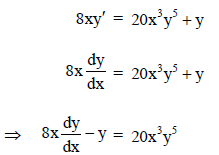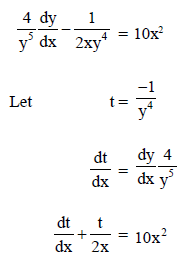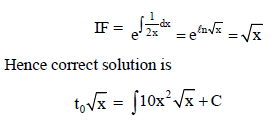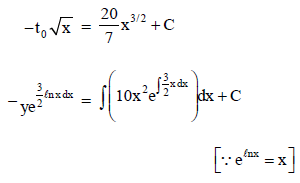*Answer can only contain numeric values
Practice Test: Civil Engineering (CE)- 5 - Question 58

While designing a rectangular beam of section size 300 × 450 mm with 50mm effective cover which is
made up of M25 concrete and reinforced with 4-20 mm diameter bars of Fe415 grade of steel. The lever
arm for the beam in limit state method of design as per IS456:2000 is

__________________mm.

*Answer can only contain numeric values
Practice Test: Civil Engineering (CE)- 5 - Question 59

A catchment area is in the form of a sector of circle of radius 3km and angle 30°. Its hydrograph can
be approximated to a traingle having time base = 12 hr. The effective rainfall during the storm of 6-hr
duration is 3cm. The peak of this hydrograph will be

_____________ m3/s

Practice Test: Civil Engineering (CE)- 5 - Question 60

If 51 mg OH is present in the water then pH of that sample is

Practice Test: Civil Engineering (CE)- 5 - Question 61

Water flows a 20 mm I.D. at a rate of 0.02 kg/s entering at 20°C. The tube is wrapped from outside
by an electric heating element that produces a uniform flux of 18 K W/m2. If the exit temperature of
water is 50°C, find the length of the pipe needed for this process. Given water properties at 35°C, r =
997 kg/m3, Cp = 4180 J/kg-K, m = 820 × 10–6 Ns/m2 and k = 0.808 W/mK

Detailed Solution for Practice Test: Civil Engineering (CE)- 5 - Question 61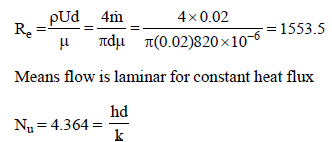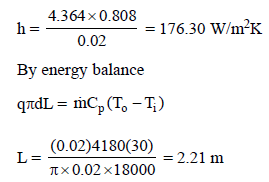Practice Test: Civil Engineering (CE)- 5 - Question 62

Find the value of λ and μ for which system of equations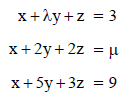has infinite consistent solution

Detailed Solution for Practice Test: Civil Engineering (CE)- 5 - Question 62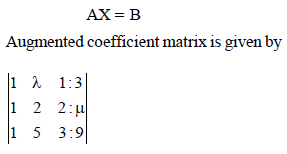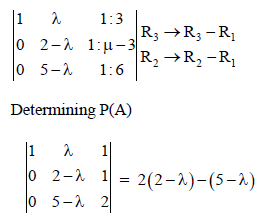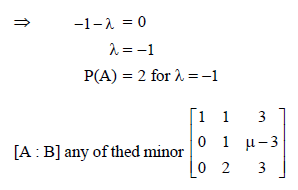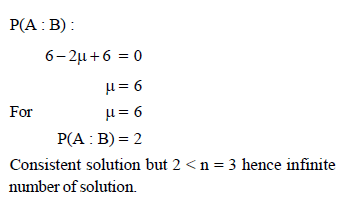Practice Test: Civil Engineering (CE)- 5 - Question 63

The total float for activity 2-4, for network shown below will be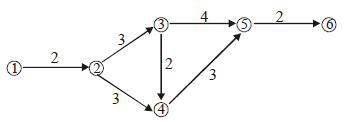*Answer can only contain numeric values
Practice Test: Civil Engineering (CE)- 5 - Question 64

An elevated structure with total weight of 10,000 kN is supported on tower with 4 legs. The legs rest on piers
located at corners of square 6m on aside. What is the vertical stress increment due to loading at a point 7m
beneath the centre of the structure is

__________________ kN/m2

Detailed Solution for Practice Test: Civil Engineering (CE)- 5 - Question 64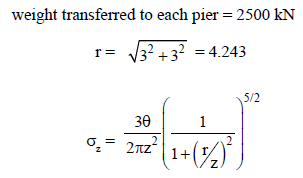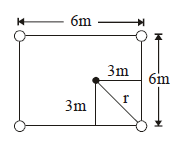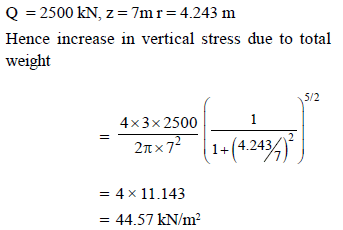*Answer can only contain numeric values
Practice Test: Civil Engineering (CE)- 5 - Question 65

Following loads are at service conditions, DL = 450 N/m, LL = 500 N/m, WL = 250 N/m, EQL = 220 N/m.
The design load for limit state of collapse is

________________ N/m

## GATE Civil Engineering (CE) 2023 Mock Test Series

27 docs|296 tests
 Use Code STAYHOME200 and get INR 200 additional OFF Use Coupon Code
Information about Practice Test: Civil Engineering (CE)- 5 Page
In this test you can find the Exam questions for Practice Test: Civil Engineering (CE)- 5 solved & explained in the simplest way possible. Besides giving Questions and answers for Practice Test: Civil Engineering (CE)- 5, EduRev gives you an ample number of Online tests for practice

## GATE Civil Engineering (CE) 2023 Mock Test Series

27 docs|296 tests